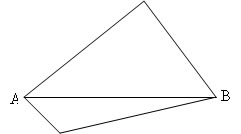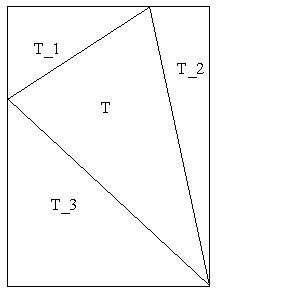#### You may also like### Areas and Ratios

Do you have enough information to work out the area of the shaded quadrilateral?### Shape and Territory

If for any triangle ABC tan(A - B) + tan(B - C) + tan(C - A) = 0 what can you say about the triangle?### Napoleon's Hat

Three equilateral triangles ABC, AYX and XZB are drawn with the point X a moveable point on AB. The points P, Q and R are the centres of the three triangles. What can you say about triangle PQR?

# Proof of Pick's Theorem

##### Age 16 to 18 Challenge Level:

Here is another excellent solution from Andrei of Tudor Vianu National College, Bucharest, Romania.

I shall prove Pick's Theorem by proving that Pick's function $$F(P)= {\rm area }(P) - (i + {p\over 2} - 1)$$ is 0 for any planar polygon, following the steps from the problem.

(1) Let $P_1$ and $P_2$ be two polygons with a common edge, $P$ the union of $P_1$ and $P_2$, $i$, $i_1$, $i_2$ the lattice points inside the 3 polygons, and $p$, $p_1$, $p_2$ the lattice points on the perimeters of the 3 polygons.

Let $x$ be the number of lattice points of the common edge of $P_1$ and $P_2$. It is clear that area($P$) = area($P_1$) + area($P_2$)From the figure I observe that: $i = i_1 + i_2 + x - 2$ $p = p_1 + p_2 - 2x + 2.$ Now, I shall calculate $F(P)$:

\begin{eqnarray} F(P)&=& {\rm area }(P) - (i + {p\over 2} - 1)\\ &=& {\rm area }(P_1)+{\rm area }(P_2)-i_1 -i_2 -x + 2 - {p_1 \over 2} - {p_2\over 2} + x - 1 + 1 \\ &=& {\rm area }(P_1)+{\rm area }(P_2)-i_1 -i_2 - {p_1\over 2} - {p_2\over 2} + 2\\ &=& {\rm area }(P_1) - (i + {p_1\over 2} - 1)+{\rm area }(P_2)- (i + {p_2\over 2} - 1)\\ &=& F(P_1) + F(P_2) \end{eqnarray} So, if Pick's function is zero for any two of these polygons it must be zero for the third. This means that if Pick's Theorem holds for any two of these polygons it must hold for the third. As a generalization, $$F(P_1+P_2+ ... +P_n) = F(P_1) + F(P_2) + ... +F(P_n).$$ So, $F$ is additive.

(2) Now I shall prove Pick's Theorem for a rectangle with vertices $(0,0), (a,0), (a,b), (0,b)$.
${\rm Area}(P) = ab$, $i = (a - 1)(b - 1)$ and $p = 2(a + b).$ So $$F(P) = ab - (a-1)(b-1) - (a+b) + 1 = 0.$$ Any rectangle with sides parallel to Ox and Oy could be translated to a rectangle of the given form.

(3) If the rectangle is split into two triangles by a diameter then each triangle has the same area and the same number of interior points and the same number of points on the perimeter and hence Pick's function must take the same value for each triangle. By parts (1) and (2) Pick's function must be zero for such triangles.

When a polygon with vertices at lattice points is translated so that the image has vertices at lattice points there is no change in area, or in the number of interior lattice points, or in the number of lattice points on the perimeter. Hence Pick's function is zero for all rectangles and for any right-angled triangle which has twosides parallel to the coordinate axes.(4) Now I shall prove the theorem for any triangle. Let $T$ be a triangle. Any shape of triangle can be enclosed in a rectangle with edges parallel to the coordinate axes, as in the diagram, by adding at most three triangles. From part (1): $$F(T+T1+T2+T3) = F(T) + F(T1) + F(T2) + F(T3).$$ But from part (2): $$F(T+T1+T2+T3) = 0$$ and from part (3): $$F(T1) = F(T2) = F(T3) = 0.$$ From these relations, I observe that $F(T) = 0$.
(5) As any polygon can be split into triangles and rectangles, the theorem is proved.